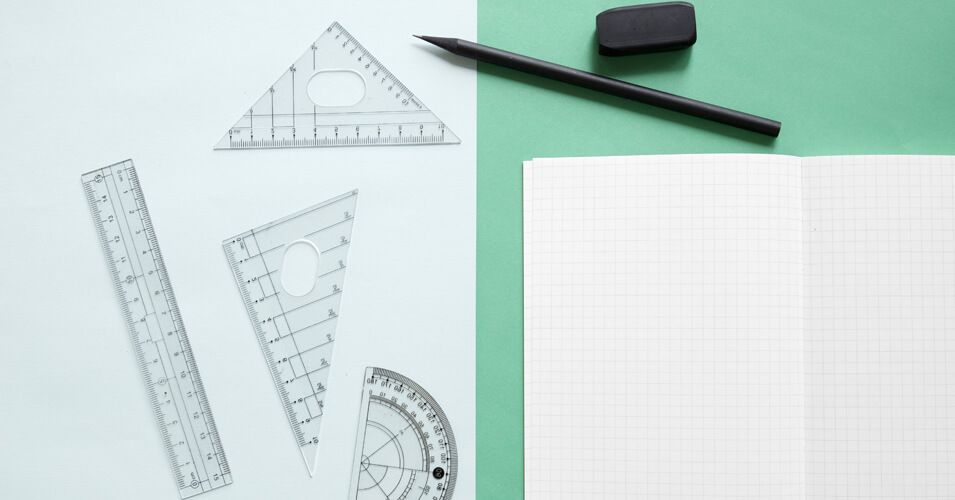• Save

# Coordinate Geometry Questions and Answers

Vikram Singh2 years ago 11.9K ViewsQ :

Find co-ordinates of the midpoint of the segment joining points C (3, -5) and D ( -7,3)

(A) (-5, 4)

(B) (2,1)

(C) (-2, -1)

(D) (5, -4)

Q :

In what ratio does the point T (x, 0) divide the segment joining the points S ( -4, -1 ) and U ( 1,4 )?

(A) 1 : 2

(B) 2 : 1

(C) 1 : 4

(D) 4 : 1

Q :

For what value of k, the system of equations kx + 2y = 2 and 3x + y = 1 will be coincident?

(A) 5

(B) 6

(C) 2

(D) 3

Q :

Reflection of the point (-1, 4) in the x-axis is:

(A) (-1, 4)

(B) (-1, -4)

(C) (1, 4)

(D) (1, -4)

Q :

If ax - 4y = -6 has a slope of -3 / 2. What is the value of a?

(A) 3

(B) -6

(C) 6

(D) -3

Q :

Find the area of quadrilateral formed by joining points (4,2), (8,2), (8,14) and (4,10).

(A) 25 Sq. units

(B) 40 Sq. units

(C) 5 Sq. units

(D) 10 Sq. units

Q :

The slopes of two lines are 1 and √3. What is the angle between these two lines?

(A) $$45^0$$

(B) $$60^0$$

(C) $$15^0$$

(D) $$30^0$$

Q :

The distance between the points (4, 8) and (k, -4) is 13. What is the value of k?

(A) -1

(B) -3

(C) 1

(D) 3

Q :

Area of the triangle formed by the graph of the straight lines x - y = 0, x + y = 2 and the x-axis is:

(A) 2 sq units

(B) 4 sq units

(C) 1 sq unit

(D) None of these

Q :

Which of the following points lies on the line 5x + 4y = 2?

(A) (-2,3)

(B) (2,-3)

(C) ( 2,3 )

(D) (-2,-3)

### Vikram Singh

Providing knowledgable questions of Reasoning and Aptitude for the competitive exams.